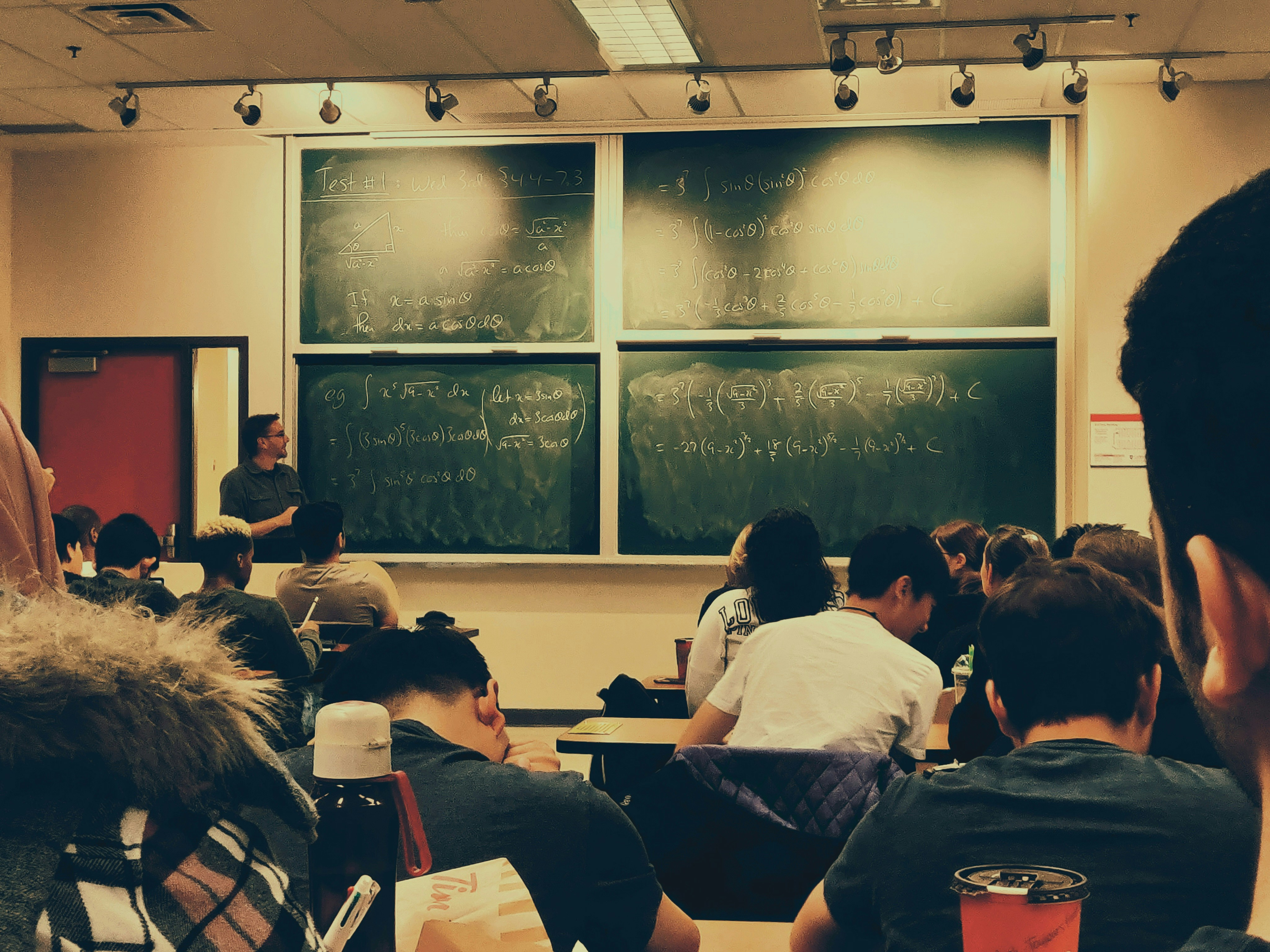# Calculus For Kids

## Learn it the easier way

By Arun RamasamyPublished 2 months ago Updated 2 months ago 3 min read
3Photo by Shubham Sharan on Unsplash

what is calculus

Calculus is a branch of mathematics that deals with the study of change and motion. It is divided into two main branches: differential calculus and integral calculus.

We see that even young adults find it difficult to understand and explain the concepts of calculus.

Let's see how better we can try to understand those concepts in a simple way.

Differential calculus is a branch of mathematics that helps us understand how things change. Imagine you have a balloon that you blow up. As you blow up the balloon, it gets bigger and bigger. The rate at which the balloon is getting bigger is called the "rate of change" or "derivative."

For example, let's say the balloon is getting bigger by 3 inches per second. We can write this as:

d(size of balloon)/dt = 3 inches/second

This equation tells us that the rate of change of the balloon's size (how fast it is getting bigger) is 3 inches per second.

Now, let's say you stop blowing up the balloon and it starts to deflate. The rate at which the balloon is getting smaller is also called the "rate of change" or "derivative."

So, if the balloon is getting smaller by 1 inch per second, we can write this as:

d(size of balloon)/dt = -1 inch/second

This equation tells us that the rate of change of the balloon's size (how fast it is getting smaller) is -1 inch per second.

Take another example, let's say you have a toy car, and you want to know how fast it is moving. You can use differential calculus to help you figure that out.

Here's an example: Let's say you start timing how long it takes for the toy car to travel a certain distance, say, 5 meters.

You notice that it takes the car 2 seconds to travel that distance. So, you can calculate the speed of the car using a formula:

Speed = distance / time

So in this case, the speed of the car is 5 meters / 2 seconds = 2.5 meters per second.

Now, let's say you want to know how the speed of the toy car is changing. You can use differential calculus to figure that out. You can measure the speed of

the car at different moments in time, and then calculate the rate of change, or "derivative" of the speed. The derivative tells you how fast the speed is changing.

Differential calculus helps us understand how things change by looking at the rate of change or the derivative.

It's like a detective work to find out how things change over time.

Integral calculus is a way to figure out how much of something there is.

It's like counting how many things you have, but instead of counting physical things, we are counting the amount of something over a certain period of time or distance.

Instead, we use mathematical formulas and techniques to determine the total amount of something.

For example, let's say you have a jar full of marbles and you want to know how many marbles there are in total. You can use integral calculus to help you figure that out.

Here's an example: let's say you want to count the marbles in the jar, but instead of counting them one by one, you use a scoop to scoop out some marbles and count how many marbles there are in the scoop.

You do this multiple times until you scoop out all the marbles. Each time you scoop out marbles, you add the number of marbles in the scoop to a tally.

The total number of marbles in the jar is the sum of all the marbles in the scoops, or the integral of the marbles.

Imagine you have a bucket full of water, and you want to know how much water is in the bucket. You can use integral calculus to help you figure that out.

Here's an example: Let's say you want to measure the amount of water in the bucket, but instead of measuring the whole bucket at once, you use a measuring cup to measure some water, and then add it to a larger container.

You do this multiple times until you measure all the water in the bucket. Each time you measure water,

you add the amount of water to a tally. The total amount of water in the bucket is the sum of all the water measured,

or the integral of the water.

On the whole calculus acts a bridge for childrens to understand the concepts of change and motion in the world around them.

It helps them to make sense of things they see happening in the world and to understand how things change over time.

Happy Solving Problems !!!!

teacherstudenthow tohigh school
3

### Arun Ramasamy

Nature Lover, Just go with the flow, techno freek.

Do what you can.. don't when you cannot.

How does it work?

There are no comments for this story

Be the first to respond and start the conversation.